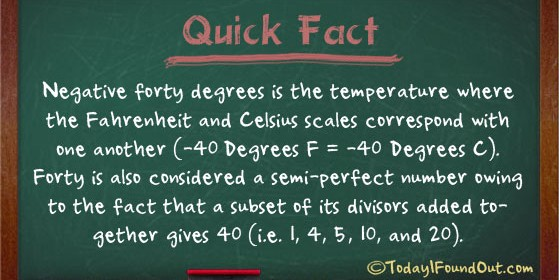## -40 Degrees is the Temperature Where the Fahrenheit and Celsius Scales Meet (-40°F = -40°C)Negative forty degrees is the temperature where the Fahrenheit and Celsius scales correspond with one another (-40°F = -40°C).  Forty is also considered a semi-perfect number owing to the fact that a subset of its divisors added together gives 40 (i.e. 1, 4, 5, 10, and 20). Reference